Open in App
Not now

# Dynamic Memory Allocation in C using malloc(), calloc(), free() and realloc()

• Difficulty Level : Easy
• Last Updated : 23 Dec, 2022

Since C is a structured language, it has some fixed rules for programming. One of them includes changing the size of an array. An array is a collection of items stored at contiguous memory locations.As it can be seen that the length (size) of the array above made is 9. But what if there is a requirement to change this length (size). For Example,

• If there is a situation where only 5 elements are needed to be entered in this array. In this case, the remaining 4 indices are just wasting memory in this array. So there is a requirement to lessen the length (size) of the array from 9 to 5.
• Take another situation. In this, there is an array of 9 elements with all 9 indices filled. But there is a need to enter 3 more elements in this array. In this case, 3 indices more are required. So the length (size) of the array needs to be changed from 9 to 12.

This procedure is referred to as Dynamic Memory Allocation in C.
Therefore, C Dynamic Memory Allocation can be defined as a procedure in which the size of a data structure (like Array) is changed during the runtime.
C provides some functions to achieve these tasks. There are 4 library functions provided by C defined under <stdlib.h> header file to facilitate dynamic memory allocation in C programming. They are:

1. malloc()
2. calloc()
3. free()
4. realloc()

Let’s look at each of them in greater detail.

### C malloc() method

The “malloc” or “memory allocation” method in C is used to dynamically allocate a single large block of memory with the specified size. It returns a pointer of type void which can be cast into a pointer of any form. It doesn’t Initialize memory at execution time so that it has initialized each block with the default garbage value initially.

Syntax:

```ptr = (cast-type*) malloc(byte-size)
For Example:```

ptr = (int*) malloc(100 * sizeof(int));
Since the size of int is 4 bytes, this statement will allocate 400 bytes of memory. And, the pointer ptr holds the address of the first byte in the allocated memory.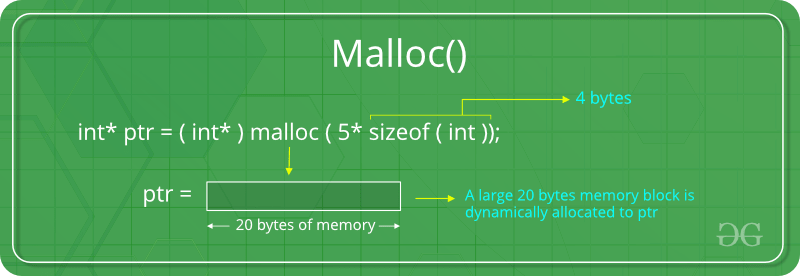If space is insufficient, allocation fails and returns a NULL pointer.

Example:

## C

 `#include ``#include `` ` `int` `main()``{`` ` `    ``// This pointer will hold the``    ``// base address of the block created``    ``int``* ptr;``    ``int` `n, i;`` ` `    ``// Get the number of elements for the array``    ``printf``(``"Enter number of elements:"``);``    ``scanf``(``"%d"``,&n);``    ``printf``(``"Entered number of elements: %d\n"``, n);`` ` `    ``// Dynamically allocate memory using malloc()``    ``ptr = (``int``*)``malloc``(n * ``sizeof``(``int``));`` ` `    ``// Check if the memory has been successfully``    ``// allocated by malloc or not``    ``if` `(ptr == NULL) {``        ``printf``(``"Memory not allocated.\n"``);``        ``exit``(0);``    ``}``    ``else` `{`` ` `        ``// Memory has been successfully allocated``        ``printf``(``"Memory successfully allocated using malloc.\n"``);`` ` `        ``// Get the elements of the array``        ``for` `(i = 0; i < n; ++i) {``            ``ptr[i] = i + 1;``        ``}`` ` `        ``// Print the elements of the array``        ``printf``(``"The elements of the array are: "``);``        ``for` `(i = 0; i < n; ++i) {``            ``printf``(``"%d, "``, ptr[i]);``        ``}``    ``}`` ` `    ``return` `0;``}`

Output:

```Enter number of elements: 5
Memory successfully allocated using malloc.
The elements of the array are: 1, 2, 3, 4, 5,```

### C calloc() method

1. “calloc” or “contiguous allocation” method in C is used to dynamically allocate the specified number of blocks of memory of the specified type. it is very much similar to malloc() but has two different points and these are:
2. It initializes each block with a default value ‘0’.
3. It has two parameters or arguments as compare to malloc().

Syntax:

```ptr = (cast-type*)calloc(n, element-size);
here, n is the no. of elements and element-size is the size of each element.```

For Example:

ptr = (float*) calloc(25, sizeof(float));
This statement allocates contiguous space in memory for 25 elements each with the size of the float.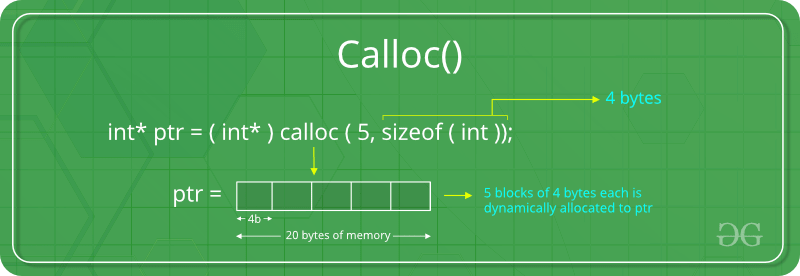If space is insufficient, allocation fails and returns a NULL pointer.

Example:

## C

 `#include ``#include `` ` `int` `main()``{`` ` `    ``// This pointer will hold the``    ``// base address of the block created``    ``int``* ptr;``    ``int` `n, i;`` ` `    ``// Get the number of elements for the array``    ``n = 5;``    ``printf``(``"Enter number of elements: %d\n"``, n);`` ` `    ``// Dynamically allocate memory using calloc()``    ``ptr = (``int``*)``calloc``(n, ``sizeof``(``int``));`` ` `    ``// Check if the memory has been successfully``    ``// allocated by calloc or not``    ``if` `(ptr == NULL) {``        ``printf``(``"Memory not allocated.\n"``);``        ``exit``(0);``    ``}``    ``else` `{`` ` `        ``// Memory has been successfully allocated``        ``printf``(``"Memory successfully allocated using calloc.\n"``);`` ` `        ``// Get the elements of the array``        ``for` `(i = 0; i < n; ++i) {``            ``ptr[i] = i + 1;``        ``}`` ` `        ``// Print the elements of the array``        ``printf``(``"The elements of the array are: "``);``        ``for` `(i = 0; i < n; ++i) {``            ``printf``(``"%d, "``, ptr[i]);``        ``}``    ``}`` ` `    ``return` `0;``}`

Output:

```Enter number of elements: 5
Memory successfully allocated using calloc.
The elements of the array are: 1, 2, 3, 4, 5,```

### C free() method

“free” method in C is used to dynamically de-allocate the memory. The memory allocated using functions malloc() and calloc() is not de-allocated on their own. Hence the free() method is used, whenever the dynamic memory allocation takes place. It helps to reduce wastage of memory by freeing it.

Syntax:

`free(ptr);`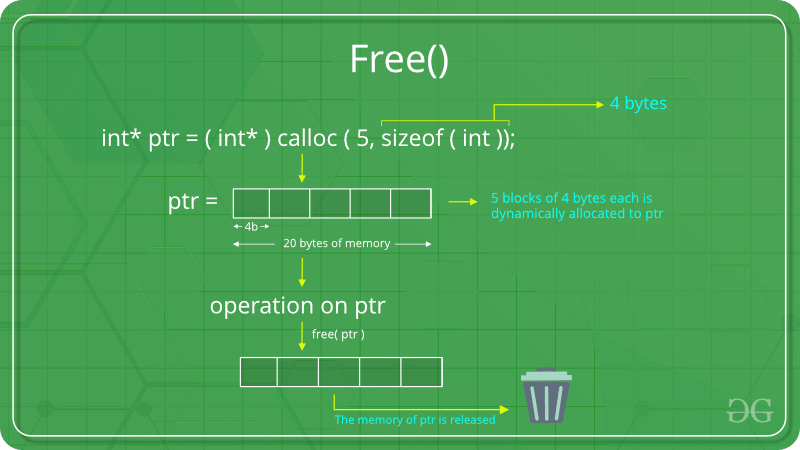Example:

## C

 `#include ``#include `` ` `int` `main()``{`` ` `    ``// This pointer will hold the``    ``// base address of the block created``    ``int` `*ptr, *ptr1;``    ``int` `n, i;`` ` `    ``// Get the number of elements for the array``    ``n = 5;``    ``printf``(``"Enter number of elements: %d\n"``, n);`` ` `    ``// Dynamically allocate memory using malloc()``    ``ptr = (``int``*)``malloc``(n * ``sizeof``(``int``));`` ` `    ``// Dynamically allocate memory using calloc()``    ``ptr1 = (``int``*)``calloc``(n, ``sizeof``(``int``));`` ` `    ``// Check if the memory has been successfully``    ``// allocated by malloc or not``    ``if` `(ptr == NULL || ptr1 == NULL) {``        ``printf``(``"Memory not allocated.\n"``);``        ``exit``(0);``    ``}``    ``else` `{`` ` `        ``// Memory has been successfully allocated``        ``printf``(``"Memory successfully allocated using malloc.\n"``);`` ` `        ``// Free the memory``        ``free``(ptr);``        ``printf``(``"Malloc Memory successfully freed.\n"``);`` ` `        ``// Memory has been successfully allocated``        ``printf``(``"\nMemory successfully allocated using calloc.\n"``);`` ` `        ``// Free the memory``        ``free``(ptr1);``        ``printf``(``"Calloc Memory successfully freed.\n"``);``    ``}`` ` `    ``return` `0;``}`

Output:

```Enter number of elements: 5
Memory successfully allocated using malloc.
Malloc Memory successfully freed.

Memory successfully allocated using calloc.
Calloc Memory successfully freed.```

### C realloc() method

“realloc” or “re-allocation” method in C is used to dynamically change the memory allocation of a previously allocated memory. In other words, if the memory previously allocated with the help of malloc or calloc is insufficient, realloc can be used to dynamically re-allocate memory. re-allocation of memory maintains the already present value and new blocks will be initialized with the default garbage value.

Syntax:

```ptr = realloc(ptr, newSize);

where ptr is reallocated with new size 'newSize'.```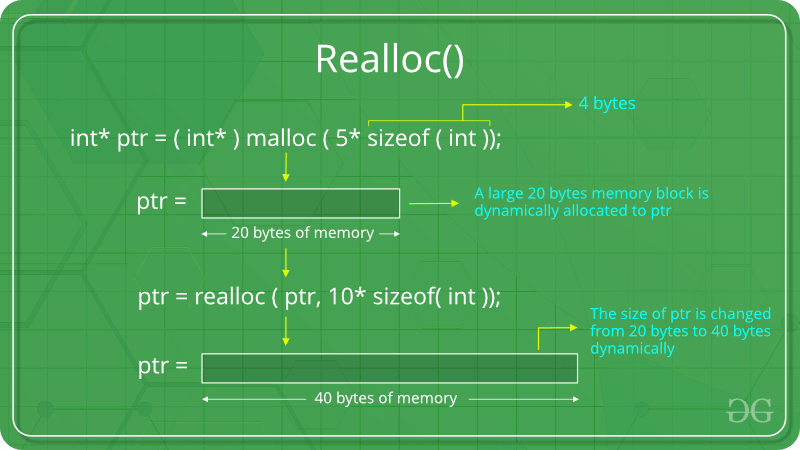If space is insufficient, allocation fails and returns a NULL pointer.

Example:

## C

 `#include ``#include `` ` `int` `main()``{`` ` `    ``// This pointer will hold the``    ``// base address of the block created``    ``int``* ptr;``    ``int` `n, i;`` ` `    ``// Get the number of elements for the array``    ``n = 5;``    ``printf``(``"Enter number of elements: %d\n"``, n);`` ` `    ``// Dynamically allocate memory using calloc()``    ``ptr = (``int``*)``calloc``(n, ``sizeof``(``int``));`` ` `    ``// Check if the memory has been successfully``    ``// allocated by malloc or not``    ``if` `(ptr == NULL) {``        ``printf``(``"Memory not allocated.\n"``);``        ``exit``(0);``    ``}``    ``else` `{`` ` `        ``// Memory has been successfully allocated``        ``printf``(``"Memory successfully allocated using calloc.\n"``);`` ` `        ``// Get the elements of the array``        ``for` `(i = 0; i < n; ++i) {``            ``ptr[i] = i + 1;``        ``}`` ` `        ``// Print the elements of the array``        ``printf``(``"The elements of the array are: "``);``        ``for` `(i = 0; i < n; ++i) {``            ``printf``(``"%d, "``, ptr[i]);``        ``}`` ` `        ``// Get the new size for the array``        ``n = 10;``        ``printf``(``"\n\nEnter the new size of the array: %d\n"``, n);`` ` `        ``// Dynamically re-allocate memory using realloc()``        ``ptr = ``realloc``(ptr, n * ``sizeof``(``int``));`` ` `        ``// Memory has been successfully allocated``        ``printf``(``"Memory successfully re-allocated using realloc.\n"``);`` ` `        ``// Get the new elements of the array``        ``for` `(i = 5; i < n; ++i) {``            ``ptr[i] = i + 1;``        ``}`` ` `        ``// Print the elements of the array``        ``printf``(``"The elements of the array are: "``);``        ``for` `(i = 0; i < n; ++i) {``            ``printf``(``"%d, "``, ptr[i]);``        ``}`` ` `        ``free``(ptr);``    ``}`` ` `    ``return` `0;``}`

Output:

```Enter number of elements: 5
Memory successfully allocated using calloc.
The elements of the array are: 1, 2, 3, 4, 5,

Enter the new size of the array: 10
Memory successfully re-allocated using realloc.
The elements of the array are: 1, 2, 3, 4, 5, 6, 7, 8, 9, 10,```

One another example for realloc() method is:

## C

 `#include ``#include ``int` `main()``{``    ``int` `index = 0, i = 0, n,``        ``*marks; ``// this marks pointer hold the base address``                ``// of  the block created``    ``int` `ans;``    ``marks = (``int``*)``malloc``(``sizeof``(``        ``int``)); ``// dynamically allocate memory using malloc``    ``// check if the memory is successfully allocated by``    ``// malloc or not?``    ``if` `(marks == NULL) {``        ``printf``(``"memory cannot be allocated"``);``    ``}``    ``else` `{``        ``// memory has successfully allocated``        ``printf``(``"Memory has been successfully allocated by "``               ``"using malloc\n"``);``        ``printf``(``"\n marks = %pc\n"``,``               ``marks); ``// print the base or beginning``                       ``// address of allocated memory``        ``do` `{``            ``printf``(``"\n Enter Marks\n"``);``            ``scanf``(``"%d"``, &marks[index]); ``// Get the marks``            ``printf``(``"would you like to add more(1/0): "``);``            ``scanf``(``"%d"``, &ans);`` ` `            ``if` `(ans == 1) {``                ``index++;``                ``marks = (``int``*)``realloc``(``                    ``marks,``                    ``(index + 1)``                        ``* ``sizeof``(``                            ``int``)); ``// Dynamically reallocate``                                   ``// memory by using realloc``                ``// check if the memory is successfully``                ``// allocated by realloc or not?``                ``if` `(marks == NULL) {``                    ``printf``(``"memory cannot be allocated"``);``                ``}``                ``else` `{``                    ``printf``(``"Memory has been successfully "``                           ``"reallocated using realloc:\n"``);``                    ``printf``(``                        ``"\n base address of marks are:%pc"``,``                        ``marks); ``////print the base or``                                ``///beginning address of``                                ``///allocated memory``                ``}``            ``}``        ``} ``while` `(ans == 1);``        ``// print the marks of the students``        ``for` `(i = 0; i <= index; i++) {``            ``printf``(``"marks of students %d are: %d\n "``, i,``                   ``marks[i]);``        ``}``        ``free``(marks);``    ``}``    ``return` `0;``}`

Output: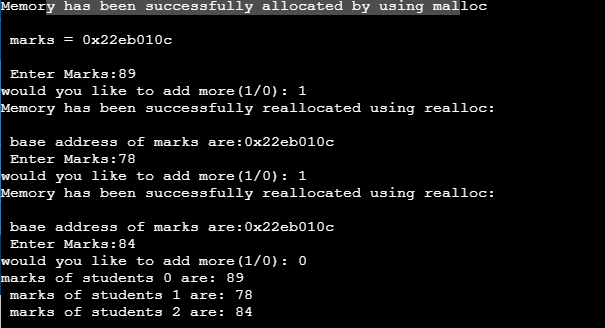My Personal Notes arrow_drop_up